## Graph rational functions

In Example 9, we see that the numerator of a rational function reveals the x-intercepts of the graph, whereas the denominator reveals the vertical asymptotes of the graph. As with polynomials, factors of the numerator may have integer powers greater than one. Fortunately, the effect on the shape of the graph at those intercepts is the same as we saw with polynomials.

The vertical asymptotes associated with the factors of the denominator will mirror one of the two toolkit reciprocal functions. When the degree of the factor in the denominator is odd, the distinguishing characteristic is that on one side of the vertical asymptote the graph heads towards positive infinity, and on the other side the graph heads towards negative infinity.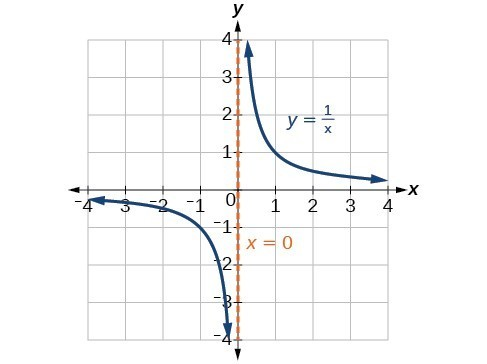Figure 17

When the degree of the factor in the denominator is even, the distinguishing characteristic is that the graph either heads toward positive infinity on both sides of the vertical asymptote or heads toward negative infinity on both sides.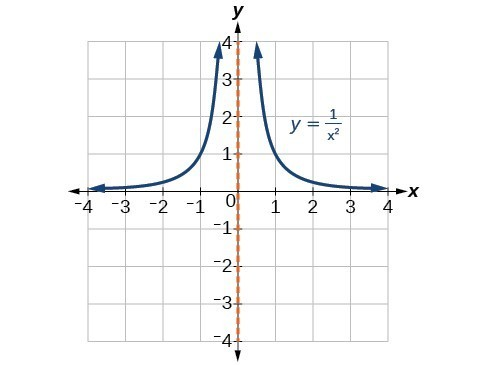Figure 18

For example, the graph of $f\left(x\right)=\frac{{\left(x+1\right)}^{2}\left(x - 3\right)}{{\left(x+3\right)}^{2}\left(x - 2\right)}$ is shown in Figure 19.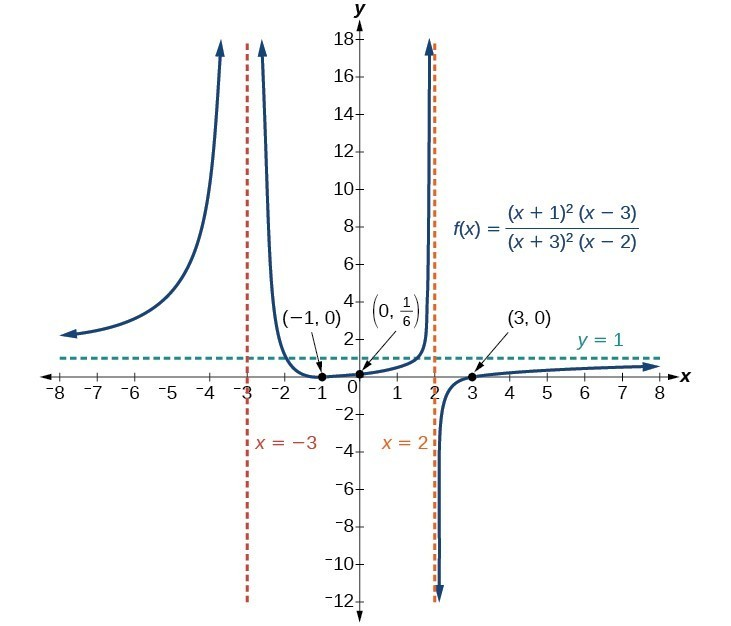Figure 19

• At the x-intercept $x=-1$ corresponding to the ${\left(x+1\right)}^{2}$ factor of the numerator, the graph bounces, consistent with the quadratic nature of the factor.
• At the x-intercept $x=3$ corresponding to the $\left(x - 3\right)$ factor of the numerator, the graph passes through the axis as we would expect from a linear factor.
• At the vertical asymptote $x=-3$ corresponding to the ${\left(x+3\right)}^{2}$ factor of the denominator, the graph heads towards positive infinity on both sides of the asymptote, consistent with the behavior of the function $f\left(x\right)=\frac{1}{{x}^{2}}$.
• At the vertical asymptote $x=2$, corresponding to the $\left(x - 2\right)$ factor of the denominator, the graph heads towards positive infinity on the left side of the asymptote and towards negative infinity on the right side, consistent with the behavior of the function $f\left(x\right)=\frac{1}{x}$.

### How To: Given a rational function, sketch a graph.

1. Evaluate the function at 0 to find the y-intercept.
2. Factor the numerator and denominator.
3. For factors in the numerator not common to the denominator, determine where each factor of the numerator is zero to find the x-intercepts.
4. Find the multiplicities of the x-intercepts to determine the behavior of the graph at those points.
5. For factors in the denominator, note the multiplicities of the zeros to determine the local behavior. For those factors not common to the numerator, find the vertical asymptotes by setting those factors equal to zero and then solve.
6. For factors in the denominator common to factors in the numerator, find the removable discontinuities by setting those factors equal to 0 and then solve.
7. Compare the degrees of the numerator and the denominator to determine the horizontal or slant asymptotes.
8. Sketch the graph.

### Example 11: Graphing a Rational Function

Sketch a graph of $f\left(x\right)=\frac{\left(x+2\right)\left(x - 3\right)}{{\left(x+1\right)}^{2}\left(x - 2\right)}$.

### Solution

We can start by noting that the function is already factored, saving us a step.

Next, we will find the intercepts. Evaluating the function at zero gives the y-intercept:

$\begin{cases}f\left(0\right)=\frac{\left(0+2\right)\left(0 - 3\right)}{{\left(0+1\right)}^{2}\left(0 - 2\right)}\hfill \\ \text{ }=3\hfill \end{cases}$

To find the x-intercepts, we determine when the numerator of the function is zero. Setting each factor equal to zero, we find x-intercepts at $x=-2$ and $x=3$. At each, the behavior will be linear (multiplicity 1), with the graph passing through the intercept.

We have a y-intercept at $\left(0,3\right)$ and x-intercepts at $\left(-2,0\right)$ and $\left(3,0\right)$.

To find the vertical asymptotes, we determine when the denominator is equal to zero. This occurs when $x+1=0$ and when $x - 2=0$, giving us vertical asymptotes at $x=-1$ and $x=2$.

There are no common factors in the numerator and denominator. This means there are no removable discontinuities.

Finally, the degree of denominator is larger than the degree of the numerator, telling us this graph has a horizontal asymptote at $y=0$.

To sketch the graph, we might start by plotting the three intercepts. Since the graph has no x-intercepts between the vertical asymptotes, and the y-intercept is positive, we know the function must remain positive between the asymptotes, letting us fill in the middle portion of the graph as shown in Figure 20.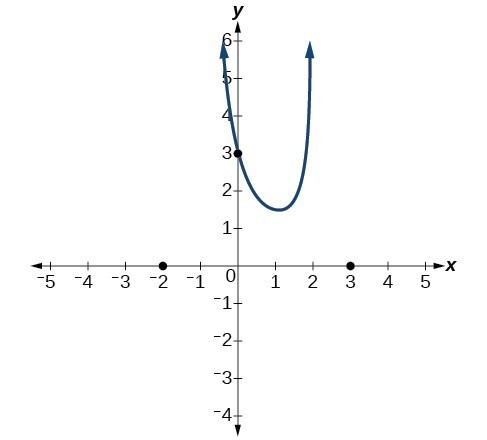Figure 20

The factor associated with the vertical asymptote at $x=-1$ was squared, so we know the behavior will be the same on both sides of the asymptote. The graph heads toward positive infinity as the inputs approach the asymptote on the right, so the graph will head toward positive infinity on the left as well.

For the vertical asymptote at $x=2$, the factor was not squared, so the graph will have opposite behavior on either side of the asymptote. After passing through the x-intercepts, the graph will then level off toward an output of zero, as indicated by the horizontal asymptote.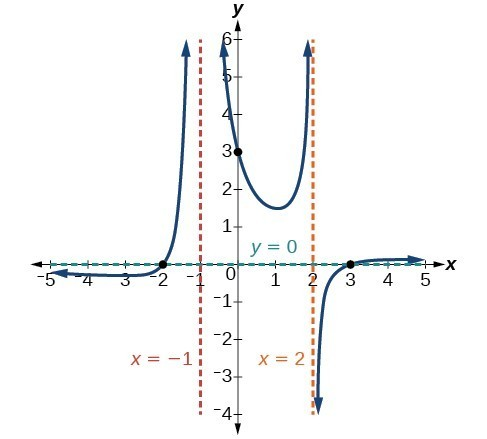Figure 21

### Try It 8

Given the function $f\left(x\right)=\frac{{\left(x+2\right)}^{2}\left(x - 2\right)}{2{\left(x - 1\right)}^{2}\left(x - 3\right)}$, use the characteristics of polynomials and rational functions to describe its behavior and sketch the function.

Solution

## Writing Rational Functions

Now that we have analyzed the equations for rational functions and how they relate to a graph of the function, we can use information given by a graph to write the function. A rational function written in factored form will have an x-intercept where each factor of the numerator is equal to zero. (An exception occurs in the case of a removable discontinuity.) As a result, we can form a numerator of a function whose graph will pass through a set of x-intercepts by introducing a corresponding set of factors. Likewise, because the function will have a vertical asymptote where each factor of the denominator is equal to zero, we can form a denominator that will produce the vertical asymptotes by introducing a corresponding set of factors.

### A General Note: Writing Rational Functions from Intercepts and Asymptotes

If a rational function has x-intercepts at $x={x}_{1}, {x}_{2}, …, {x}_{n}$, vertical asymptotes at $x={v}_{1},{v}_{2},\dots ,{v}_{m}$, and no ${x}_{i}=\text{any }{v}_{j}$, then the function can be written in the form:

$f\left(x\right)=a\frac{{\left(x-{x}_{1}\right)}^{{p}_{1}}{\left(x-{x}_{2}\right)}^{{p}_{2}}\cdots {\left(x-{x}_{n}\right)}^{{p}_{n}}}{{\left(x-{v}_{1}\right)}^{{q}_{1}}{\left(x-{v}_{2}\right)}^{{q}_{2}}\cdots {\left(x-{v}_{m}\right)}^{{q}_{n}}}$

where the powers ${p}_{i}$ or ${q}_{i}$ on each factor can be determined by the behavior of the graph at the corresponding intercept or asymptote, and the stretch factor can be determined given a value of the function other than the x-intercept or by the horizontal asymptote if it is nonzero.

### How To: Given a graph of a rational function, write the function.

1. Determine the factors of the numerator. Examine the behavior of the graph at the x-intercepts to determine the zeroes and their multiplicities. (This is easy to do when finding the “simplest” function with small multiplicities—such as 1 or 3—but may be difficult for larger multiplicities—such as 5 or 7, for example.)
2. Determine the factors of the denominator. Examine the behavior on both sides of each vertical asymptote to determine the factors and their powers.
3. Use any clear point on the graph to find the stretch factor.

### Example 12: Writing a Rational Function from Intercepts and Asymptotes

Write an equation for the rational function shown in Figure 22.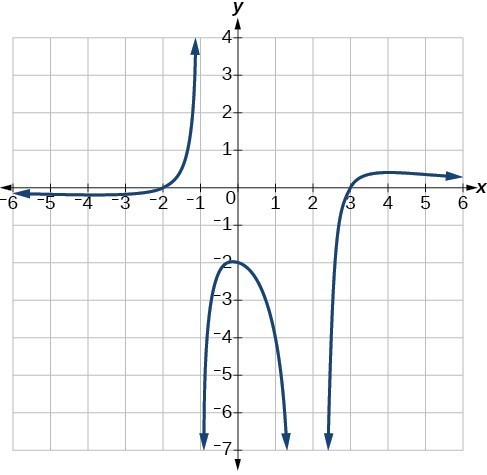Figure 22

### Solution

The graph appears to have x-intercepts at $x=-2$ and $x=3$. At both, the graph passes through the intercept, suggesting linear factors. The graph has two vertical asymptotes. The one at $x=-1$ seems to exhibit the basic behavior similar to $\frac{1}{x}$, with the graph heading toward positive infinity on one side and heading toward negative infinity on the other. The asymptote at $x=2$ is exhibiting a behavior similar to $\frac{1}{{x}^{2}}$, with the graph heading toward negative infinity on both sides of the asymptote.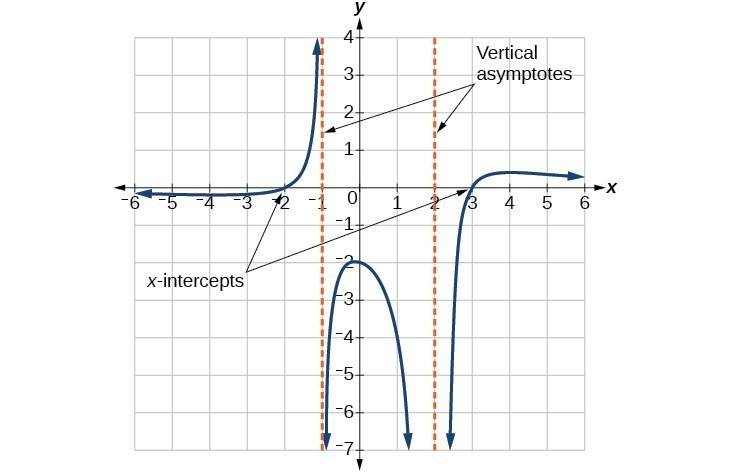Figure 23

We can use this information to write a function of the form

$f\left(x\right)=a\frac{\left(x+2\right)\left(x - 3\right)}{\left(x+1\right){\left(x - 2\right)}^{2}}$.

To find the stretch factor, we can use another clear point on the graph, such as the y-intercept $\left(0,-2\right)$.

$\begin{cases}-2=a\frac{\left(0+2\right)\left(0 - 3\right)}{\left(0+1\right){\left(0 - 2\right)}^{2}}\hfill \\ -2=a\frac{-6}{4}\hfill \\ \text{ }a=\frac{-8}{-6}=\frac{4}{3}\hfill \end{cases}$

This gives us a final function of $f\left(x\right)=\frac{4\left(x+2\right)\left(x - 3\right)}{3\left(x+1\right){\left(x - 2\right)}^{2}}$.